# How to Sum with Criteria and Or Logic in Excel

To add numbers together we need to apply SUM function. And if we want to add numbers based on some conditions, we can add criteria with the help of SUMIFS function, SUMIFS can filter data with multiple criteria effectively. If we want to filter data based on multiple criteria with OR logic, we can add array constant to help us. In this article, we will introduce you the syntax, arguments of SUM and SUMIFS function, and let you know how this formula works to reach your goal. After reading the article, you may have a simple understanding the two functions and array constant construction.

## EXAMPLE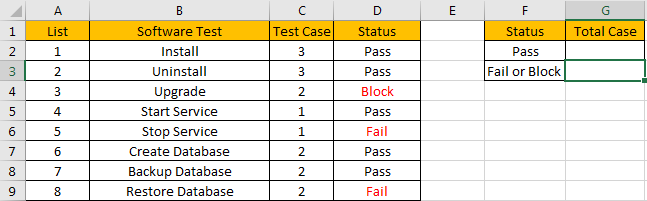Refer to the left-hand side table, we can see some tasks in software test progress are listed, for each task, there are several related test cases, for example, for task “Install”, there are 3 test cases assigned to it. In “Status” column, there are three status “Pass”, “Fail” and “Block”. For the tasks which status is “Fail” or “Block”, we need to re-test the related test cases, so we need to know how many test cases we need to select and execute in next regression test. Now, we need to calculate the re-run test case number based on the criteria “status=fail or block”, record the result in the right-hand side table “Total case” column accordingly.

In this instance, we need to pick up numbers from fail or block status, then sum them together. To fix this issue by formula, we can apply SUMIFS and SUM functions here.

## FORMULA – SUM & SUMIFS FUNCTIONS

Step 1: In G2, enter the formula

`=SUM(SUMIFS(C2:C9,D2:D9,{"Block","Fail"})).`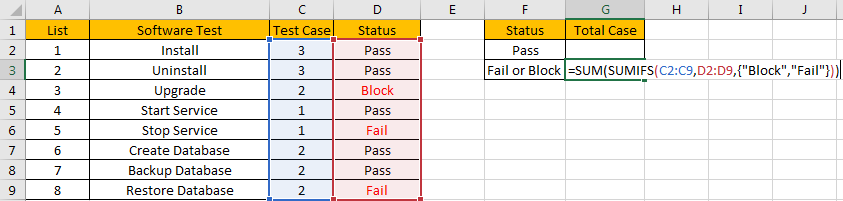Step 2: Press Enter after typing the formula.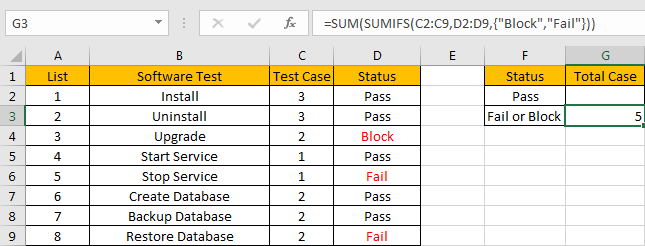We can see in column D, cell D3, D5 and D8 meet our criteria, so we just need to calculate case numbers from C3, C5 and C8, so the total case number is 2+1+2=5. The formula works correctly.

## FUNCTION INTRODUCTION

SUM function can add numbers together. It adds all supplied values from a range or a formula.

Syntax:

`=SUM (number1, [number2], [number3], ...)`

SUMIFS function can be seen as SUM+IFS, it can handle multiple ‘criteria range’ and ‘criteria’ combinations.

Syntax:

=SUMIFS(sum_range, criteria_range1, criteria1, [criteria_range2, criteria2], …)

[] part can be omitted.

For both SUM and SUMIFS function, they support wildcards like asterisk ‘*’ and question mark ‘?’, also support logical operators within its arguments. If wildcards or logical operators are required, they should be enclosed into double quotes (““).

The usage of wildcards:

1. An asterisk (*) means one or more characters.
2. A question mark (?) means one character.
3. The position of asterisk or question mark means the character(s) position relative to the entered part.

The usage of logical operators:

1. “>” – greater than
2. “<” – less than
3. “<>” – not equal to

### ALL ARGUMENTS

In this case, SUMIFS is included in SUM formula, it is the only argument of SUM function, its returned values should be accumulated by SUM function.

SUMIFS – SUM RANGE

In our instance, C2:C9 is the ‘sum range’. Test case numbers are listed in this field.

In the formula bar, select ‘C2:C9’, press F9, values in this range are expanded in an array.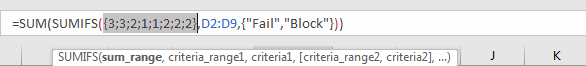SUMIFS – CRITERIA RANGE 1

D2:D9 is the criteria range. In this instance we have only one criteria range. We have only three status “Pass”, “Fail” and “Block”.

In the formula bar, select D2:D9, pree F9, values in this range are expanded in an array.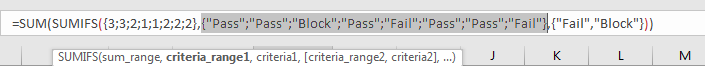SUMIFS – CRITERIA 1

As we want to calculate total test case number for tasks which “status=fail” or “status=block”, so as long as one condition is met, test case number will be recorded and accumulated. So, we will supply two criteria “Fail” and “Block” in this case, they are expanded saved in one array, a comma is added between them to split the two criteria. As SUMIFS function supports texts and wildcards, but they should be enclosed into double quotes “”, so for criteria 1, we enter {“Fail”,”Block”} finally.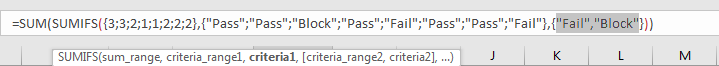Actually, the two criteria can be split to two “criteria1” in two different SUMIFS formulas, for example, if we enter =SUM(SUMIFS(C2:C9,D2:D9,”Fail”),SUMIFS(C2:C9,D2:D9,”Block”)) in G2, we can get the same result. This formula is clear but looks complex, so we use an array constant with two elements to shorten the formula.

### HOW THE FORMULA WORKS

After explaining each argument in the formula, now we will show you how the formula works with these arguments.

After expanding values in each range reference, in the formula bar, the formula is displayed as:

`=SUM(SUMIFS({3;3;2;1;1;2;2;2},{"Pass";"Pass";"Block";"Pass";"Fail";"Pass";"Pass";"Fail"},{"Fail","Block"})`

If value from the criteria range can match either value in criteria collection, “True” will be recorded in this position, otherwise, “False” will be saved instead.

{“Pass”;”Pass”;”Block”;”Pass”;”Fail”;”Pass”;”Pass”;”Fail”} – Criteria Range

{“Fail”,”Block”} – Criteria Collection

So, after comparing, we can get two new arrays based on different criteria:

{False;False;False;False;True;False;False;True} – for criteria “Fail”

{False;False;True;False;False;False;False;False} – for criteria “Block”

For the following logical operation, “True” is coerced to ‘1’ and ‘False’ is coerced to ‘0’. So above arrays are converted to two arrays only contain numbers “1” and “0”.

{0;0;0;0;1;0;0;1} – for criteria “Fail”

{0;0;1;0;0;0;0;0} – for criteria “Block”

Now, we have below two pairs of arrays:

{3;3;2;1;1;2;2;2} – sum_range

{0;0;0;0;1;0;0;1} – for criteria “Fail”

{3;3;2;1;1;2;2;2} – sum_range

{0;0;1;0;0;0;0;0} – for criteria “Block”

In the two groups, for the elements in the same position (in vertical alignment), multiply the two elements. Then we can get two new arrays.

{0;0;0;0;1;0;0;2}

{0;0;2;0;0;0;0;0}

Add all products in above two arrays separately, we get 3 and 2, actually, SUMIFS function will return {3,2} after calculation.

Now, the SUM function will add the two numbers together, that’s why the formula finally returns correct result 5. You can verify this before expanding values in the formula bar, for example, in the formula bar, select the whole formula SUMIFS(C2:C9,D2:D9,{“Fail”,”Block”}), then press F9, you can get =SUM({3,2}) in the formula bar.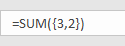1.In this case, if SUM function is omitted, we will get incorrect result, only 3 is displayed.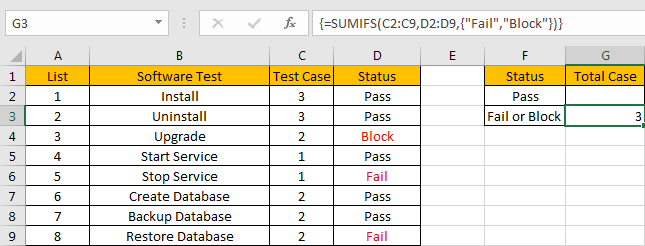In fact, in this case SUMIFS function only returns an array contains two elements, it cannot add the two elements together, so we need to add a SUM function to add them.

2. If we want to add more criteria in this case, for example except status, we also add criteria “Software Test=Start Service” or “Software Test=Stop Service”, then we can add new criteria range and new criteria.

Enter

`=SUM(SUMIFS(C2:C9,D2:D9,{"Fail","Block"},B2:B9,{"Start Service";"Stop Service"}))`

into G2, formula returns 1 as result.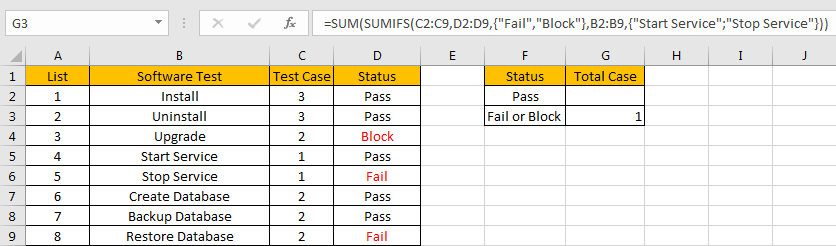In this formula, we can see that in the first array constant, a comma is displayed between the two values, but in the second array constant, a semi-colon is displayed instead of a comma. That’s because the two criteria are expanded in two array constants, each array contains two elements, to filter data based on the two criteria, we need one of them as a row array, and the other one as a column array, then after executing the formula, it will return a two dimensional array.

SUMMARY

1. SUMIFS function can handle multiple groups of criteria ranges and criteria. Sum range is the first argument among all arguments.

2. It supports user defined range name.

3. It supports wildcard.

4. It supports logical operators.

### Related Functions

• Excel SUMIF Function
The Excel SUMIF function sum the numbers in the range of cells that meet a single criteria that you specify. The syntax of the SUMIF function is as below:=SUMIF (range, criteria, [sum_range])…
• Excel SUMIFS Function
The Excel SUMIFS function sum the numbers in the range of cells that meet a single or multiple criteria that you specify. The syntax of the SUMIFS function is as below:=SUMIFS (sum_range, criteria_range1, criteria1, [criteria_range2, criteria2], …)…
• Excel SUM function
The Excel SUM function will adds all numbers in a range of cells and returns the sum of these values. You can add individual values, cell references or ranges in excel.The syntax of the SUM function is as below:= SUM(number1,[number2],…)